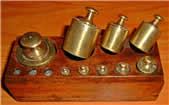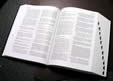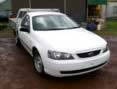# Metric Mass (Weight)Mass: how much matter is in an object.

We measure mass by weighing, but Weight and Mass are not really the same thing.

These are the most common measurements:

• Grams
• Kilograms
• Tonnes

Grams are the smallest, Tonnes are the biggest.

Let’s take a few minutes and explore how heavy each of these are.

### GramsA paperclip weighs about 1 gram. Hold one small paperclip in your hand. Does that weigh a lot? No! A gram is very light. That is why you often see things measured in hundreds of grams.

Grams are often written as g (for short), so "300 g" means "300 grams".

A loaf of bread weighs about 700 g (for a nice sized loaf)

### Kilograms

Once you have 1.000 grams, you have 1 kilogram.

 1 kilogram = 1.000 grams A dictionary has a mass of about one kilogram.Kilograms are great for measuring things that can be lifted by people (sometimes very strong people are needed of course!).Kilograms are often written as kg (that is a "k" for "kilo" and a "g" for "gram), so "10 kg" means "10 kilograms". When you weigh yourself on a scale, you would use kilograms. An adult weighs about 70 kg. How much do you weigh?

But when it comes to things that are very heavy, we need to use the tonne.

### Tonne

Once you have 1.000 kilograms, you will have 1 tonne.

1 tonne = 1.000 kilogramsTonnes (also called Metric Tons) are used to measure things that are very heavy. Things like cars, trucks and large cargo boxes are weighed using the tonne. This car weighs about 2 tonnes.

Tonnes are often written as t (for short), so "5 t" means "5 tonnes".

1 kilogram = 1.000 grams

1 tonne = 1.000 kilograms

## Weight or Mass?

We have used the word "weight" only because that is what people commonly say.

But we really should say "Mass". See Weight or Mass to learn more.

## More Examples

• a quarter of a teaspoon of sugar
• a cubic centimeter of water
• a paperclip
• a pen cap
• a thumbtack
• a pinch of salt
• a piece of gum
• the weight of any US bill
• 0,035274 of an ounce to 6 decimal places (you need 28,349523 grams to make an ounce)
• one fifth of a piece of paper (80 gsm A4 paper weighs 4,8 g)